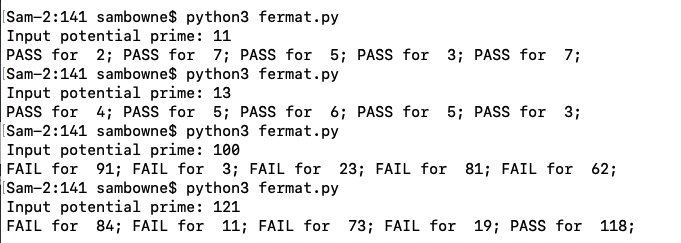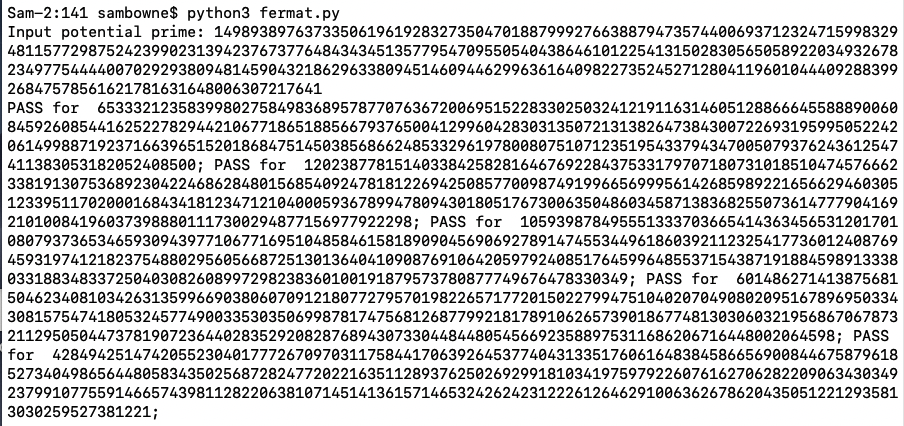# C 503: Finding Large Primes

What you need:
• Any computer with Python 3.

## Purpose

To implement the Fermat primality test and find large primes.

## Background

Fermat's little theorem states that if p is prime and a is not divisible by p, then:So testing that condition for several random values of a can be used as evidence that p is probably prime.

This site explains the Fermat primality test and its flaws in more detail:

## Simple Python Implementation

Create a file named fermat.py containing this code:
```from random import randint p = int(input("Input potential prime: ")) for i in range(5): a = randint(2,p-2) if pow(a, p-1, p) == 1: print("PASS for ", a, end="; ") else: print("FAIL for ", a, end="; ") print() ```
Run the file with this command:
```python3 fermat.py ```

## Demonstration

Try these values: 11, 13, 100, 121.

As shown below, 11 and 13 pass all the tests because they are prime, but 100 and 121 fail because they aren't.## Testing Large Numbers

Test this number, which is 1024 bits long, and is prime:

149893897637335061961928327350470188799927663887947357440069371232471599832948115772987524239902313942376737764843434513577954709550540438646101225413150283056505892203493267823497754444007029293809481459043218629633809451460944629963616409822735245271280411960104440928839926847578561621781631648006307217641

It works, because Python really can handle any size of integer, as shown below.# C 503.1: Find the Next Prime (10 pts)

This number is not prime:

10**300

Find the first number above it which is prime.

The flag is that number as one long integer, like

11111111111111111111111111111111

# C 503.2: Companion Primes (10 pts extra)

11 and 13 are both primes, only differing by two. Such primes are called companion primes.

Find the first set of companion primes above

10**100

The flag is the lower of the two primes, as one long integer, like

11111111111111111111111111111111

Posted 11-1-17 by Sam Bowne
Added to Crypto Hero 4-16-18 6:26 am
Ported to new scoring engine 7-8-19
Updated to python 3 11-5-20